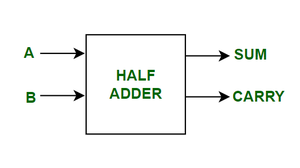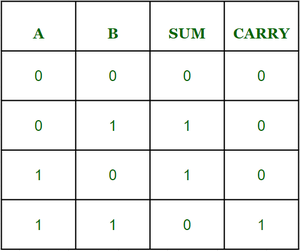# C++ program to implement Half Adder

• Difficulty Level : Medium
• Last Updated : 26 Aug, 2021

Prerequisite : Half Adder in Digital Logic

We are given with two inputs A and B. Our task is to implement Half Adder circuit and print the outputs sum and carry of two inputs.

Introduction :

The Half adder is a combinational circuit which add two1-bit binary numbers which are augend and addend to give the output value along with the carry.  The half adder has two input states and two output states. The two outputs are Sum and Carry.Here we have two inputs A, B and two outputs sum, carry. And the truth table for Half Adder isLogical Expression :

Sum = A XOR B

Carry = A AND B

Examples :

Input : A=0, B= 0

Output: Sum=0, Carry=0

Explanation:  Here from logical expression Sum = A XOR B i.e  0 XOR 0 = 0, and Carry=A AND B  i.e 0 AND 0 = 0.

Input : A=1, B= 0

Output: Sum=1, Carry=0

Explanation:   Here from  logical expression Sum=A XOR B  i.e 1 XOR 0 =1 , Carry=A AND B  i.e 1 AND 0 =  0.

Approach :

• Initialize the variables Sum and Carry for storing outputs.
• First we will take two inputs A and B.
• By applying  A XOR B we get the value of Sum.
• By applying  A AND B we get the value of Carry.

## C++

 `// C++ program for above approach ` `#include ` `using` `namespace` `std; ` `// Function to print Sum and Carry ` `void` `Half_Adder(``int` `A,``int` `B){ ` `    ``//initialize the variables Sum and Carry ` `    ``int` `Sum , Carry; ` `   `  `    ``// Calculating value of sum by applying A XOR B ` `    ``Sum = A ^ B; ` `   `  `    ``// Calculating value of Carry by applying A AND B ` `    ``Carry = A & B; ` `   `  `    ``// printing the values ` `    ``cout<<``"Sum = "``<< Sum << endl; ` `    ``cout<<``"Carry = "``<

Output

```Sum = 1
Carry = 0```
My Personal Notes arrow_drop_up
Recommended Articles
Page :